There is one equation every retail store, call center, traffic, airport or hospital manager should know by heart.  No, it’s not E = mc².  The one I had in mind is this: W = 1 / (μ – λ) It may not look like much, but it can mean the [...]

There is one equation every retail store, call center, traffic, airport or hospital manager should know by heart.  No, it’s not E = mc².  The one I had in mind is this: W = 1 / (μ – λ) It may not look like much, but it can mean the [...]

Burger and fries, wine and cheese, peanut butter and jelly … some things just go better together. For organizations embarking on digital transformation, AI and IoT just go better together. These two distinct technologies; AI and IoT (or AIoT) are a natural fit. To take an analogy from the human [...]

When solving optimization problems, it is harder to specify a constrained optimization than an unconstrained one. A constrained optimization requires that you specify multiple constraints. One little typo or a missing minus sign can result in an infeasible problem or a solution that is unrelated to the true problem. This article shows two ways to check that you've correctly specified the constraints for a two-parameter optimization. The first is a program that translates the linear constraint matrix in SAS/IML software into a system of linear equations in slope-intercept form. The second is a visualization of the constraint region.

For simplicity, this article only discusses two-dimensional constraint regions. For these regions, the SAS/IML constraint matrix is a k x 4 matrix. We also assume that the constraints are linear inequalities that define a 2-D feasible region.

The SAS/IML matrix for boundary and linear constraints

In SAS/IML software you must translate your boundary and linear constraints into a matrix that encodes the constraints. (The OPTMODEL procedure in SAS/OR software uses a more natural syntax to specify constraints.) The first and second rows of the constraint matrix specify the lower and upper bounds, respectively, for the variables. The remaining rows specify the linear constraints. In general, when you have p variables, the first p columns contain a matrix of coefficients; the (p+1)st column encodes whether the constraint is an equality or inequality; the (p+2)th column specifies the right-hand side of the linear constraints. In this article, p = 2.

The following matrix is similar to the one used in a previous article about how to find an initial guess in a feasible region. The matrix encodes three inequality constraints for a two-parameter optimization. The first two rows of the first column specify bounds for the first variable: 0 ≤ x ≤ 10. The first two rows of the second column specify bounds for the second variable: 0 ≤ y ≤ 8. The third through fifth rows specify linear inequality constraints, as indicated in the comments. The third column encodes the direction of the inequality constraints. A value of -1 means "less than"; a value of +1 means "greater than." You can also use the value 0 to indicate an equality constraint.

```proc iml; con = { 0 0 . ., /* lower bounds */ 10 8 . ., /* upper bounds */ 3 -2 -1 10, /* 3*x1 + -2*x2 LE 10 */ 5 10 -1 56, /* 5*x1 + 10*x2 LE 56 */ 4 2 1 7 }; /* 4*x1 + 2*x2 GE 7 */```

Verify the constraint matrix

A pitfall of encoding the constraints is that you might wonder whether you made a mistake when you typed the constraint matrix. Also, each linear constraint (row) in the matrix represents a linear equation in "standard form" such as 3*x - 2*y ≤ 10, whereas you might be more comfortable visualizing constraints in the equivalent "slope-intercept form" such as "y ≥ (3/2)x - 5.

It is easy to transform the equations from standard form to slope-intercept form. If c2 > 0, then the standard equation c1*x + c2*y ≥ c0 is transformed to y ≥ (-c1/c2)*x + (c0/c2). If c2 < 0, you need to remember to reverse the sign of the inequality: y ≤ (-c1/c2)*x + (c0/c2). Because the (p+1)th column has values ±1, you can use the SIGN function to perform all transformations without writing a loop. The following function "decodes" the constraint matrix and prints it is "human readable" form:

```start BLCToHuman(con); nVar = ncol(con) - 2; Range = {"x", "y"} + " in [" + char(con[1,1:nVar]`) + "," + char(con[2,1:nVar]`) + "]"; cIdx = 3:nrow(con); /* rows for the constraints */ c1 = -con[cIdx,1] / con[cIdx,2]; c0 = con[cIdx,4] / con[cIdx,2]; sign = sign(con[cIdx,3] # con[cIdx,2]); s = {"<=" "=" ">="}; idx = sign + 2; /* convert from {-1 0 1} to {1 2 3} */ EQN = "y" + s[idx] + char(c1) + "x + " + char(c0); print Range, EQN; finish;   run BLCToHuman(con);```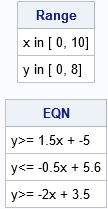Visualize the feasible region

In a previous article, I showed a technique for visualizing a feasible region. The technique is crude but effective. You simply evaluate the constraints at each point of a dense, regular, grid. If a point satisfies the constraints, you plot it in one color; otherwise, you plot it in a different color.

For linear constraints, you can perform this operation by using matrix multiplication. If A is the matrix of linear coefficients and all inequalities are "greater than" constraints, then you simply check that A*X ≥ b, where b is the right-hand side (the (p+2)th column). If any of the inequalities are "less than" constraints, you can multiply that row of the constraint matrix by -1 to convert it into an equivalent "greater than" constraint. This algorithm is implemented in the following SAS/IML function:

```start PlotFeasible(con); L = con[1, 1:2]; /* lower bound constraints */ U = con[2, 1:2]; /* upper bound constraints */ cIdx = 3:nrow(con); /* rows for linear inequality constraints */ C = con[cIdx,] # con[cIdx,3]; /* convert all inequalities to GT */ M = C[, 1:2]; /* coefficients for linear constraints */ RHS = C[, 4]; /* right hand side */ x = expandgrid( do(L, U, (U-L)/50), /* define (x,y) grid from bounds */ do(L, U, (U-L)/50) ); q = (M*x`>= RHS); /* 0/1 indicator matrix */ Feasible = (q[+,] = ncol(cIdx)); /* are all constraints satisfied? */ call scatter(x[,1], x[,2],) group=Feasible grid={x y} option="markerattrs=(symbol=SquareFilled)"; finish;   ods graphics / width=430px height=450px; title "Feasible Region"; title2 "Formed by Bounds and Linear Constraints"; run PlotFeasible(con);```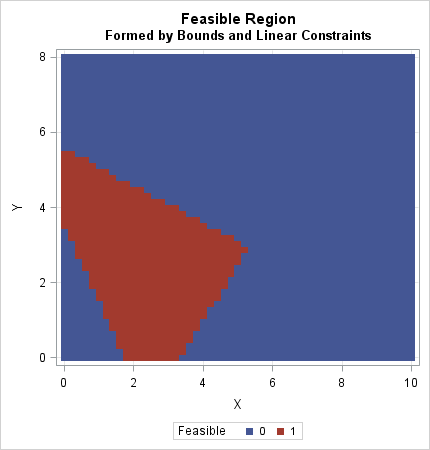The graph shows that the feasible region as a red pentagonal region. The left and lower edges are determined by the bounds on the parameters. There are three diagonal lines that are determined by the linear inequality constraints. By inspection, you can see that the point (2, 2) is in the feasible region. Similarly, the point (8,6) is in the blue region and is not feasible.

The technique uses the bounds on the parameters (the first two rows) to specify the range of the axes in the plot. If your problem does not have explicit upper/lower bounds on the parameters, you can "invent" bounds such as [0, 100] or [-10, 10] just for plotting the feasible region.

In summary, this article shows two techniques that can help you verify that a constraint matrix is correctly specified. The first translates that constraint matrix into "human readable" form. The second draws a crude approximation of the feasible region by evaluating the constraints at each location on a dense grid. Both techniques are shown for two-parameter problems. However, with a little effort, you could incorporate the second technique when searching for a good initial guess in higher-dimensional problems.

The post Visualize the feasible region for a constrained optimization appeared first on The DO Loop.This article shows how to perform an optimization in SAS when the parameters are restricted by nonlinear constraints. In particular, it solves an optimization problem where the parameters are constrained to lie in the annular region between two circles. The end of the article shows the path of partial solutions which converges to the solution and verifies that all partial solutions lie within the constraints of the problem.

The four kinds of constraints in optimization

The term "nonlinear constraints" refers to bounds placed on the parameters. There are four types of constraints in optimization problems. From simplest to most complicated, they are as follows:

• Unconstrained optimization: In this class of problems, the parameters are not subject to any constraints.
• Bound-constrained optimization: One or more parameters are bounded. For example, the scale parameter in a probability distribution is constrained by σ > 0.
• Linearly constrained optimization: Two or more parameters are linearly bounded. In a previous example, I showed images of a polygonal "feasible region" that can result from linear constraints.
• Nonlinearly constrained optimization: Two or more parameters are nonlinearly bounded. This article provides an example.

A nonlinearly constrained optimization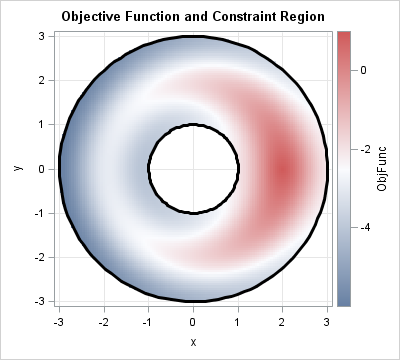This article solves a two-dimensional nonlinearly constrained optimization problem. The constraint region will be the annular region defined by the two equations
x2 + y2 ≥ 1
x2 + y2 ≤ 9

Let f(x,y) be the objective function to be optimized. For this example,
f(x,y) = cos(π r) - Dist( (x,y), (2,0) )
where
r = sqrt(x2 + y2) and
Dist( (x,y), (2,0) ) = sqrt( (x-2)2 + (y-0)2 )
is the distance from (x,y) to the point (2,0).

By inspection, the maximum value of f occurs at (x,y) = (2,0) because the objective function obtains its largest value (1) at that point. The following SAS statements use a scatter plot to visualize the value of the objective function inside the constraint region. The graph is shown to the right.

```/* evaluate the objective function on a regular grid */ data FuncViz; do x = -3 to 3 by 0.05; do y = -3 to 3 by 0.05; r2 = x**2 + y**2; r = sqrt(r2); ObjFunc = cos(constant('pi')*r) - sqrt( (x-2)**2+(y-0)**2 ); if 1 <= r2 <= 9 then output; /* output points in feasible region */ end; end; run;   /* draw the boundary of the constraint region */ %macro DrawCircle(R); R = &R; do t = 0 to 2*constant('pi') by 0.05; xx= R*cos(t); yy= R*sin(t); output; end; %mend; data ConstrBoundary; %DrawCircle(1) %DrawCircle(3) run;   ods graphics / width=400px height=360px antialias=on antialiasmax=12000 labelmax=12000; title "Objective Function and Constraint Region"; data Viz; set FuncViz ConstrBoundary; run;   proc sgplot data=Viz noautolegend; xaxis grid; yaxis grid; scatter x=x y=y / markerattrs=(symbol=SquareFilled size=4) colorresponse=ObjFunc colormodel=ThreeColorRamp; polygon ID=R x=xx y=yy / lineattrs=(thickness=3); gradlegend; run;```

Solving a constrained optimization problem in SAS/IML

SAS/IML and SAS/OR each provide general-purpose tools for optimization in SAS. This section solves the problem by using SAS/IML. A solution that uses PROC OPTMODEL is more compact and is shown at the end of this article.

SAS/IML supports 10 different optimization algorithms. All support unconstrained, bound-constrained, and linearly constrained optimization. However, only two support nonlinear constraints: the quasi-Newton method (implemented by using the NLPQN subroutine) and the Nelder-Mead simplex method (implemented by using the NLPNMS subroutine).

To specify k nonlinear constraints in SAS/IML, you need to do two things:

• Use the options vector to the NLP subroutines to specify the number of nonlinear constraints. Set optn = k to tell SAS/IML that there are a total of k constraints. If any of the constraints are equality constraints, specify that number in the optn field. That is, if there are keq equality constraints, then optn = keq.
• Write a SAS/IML module that accepts a parameter value as input and produces a column vector that contains k rows. Each row representing the evaluation of one of the constraints on the input parameters. For consistency, all nonlinear inequality constraints should be written in the form g(c) ≥ 0. The first keq rows contains the equality constraints and the remaining kkeq elements contain the inequality constraints. You specify the module that evaluates the nonlinear constraints by using the NLC= option on the NLPQN or NLPNMS subroutine.

For the current example, k = 2 and keq = 0. Therefore the module that specifies the nonlinear constraints should return two rows. The first row evaluates whether a parameter value is outside the unit circle. The second row evaluates whether a parameter value is inside the circle with radius 3. Both constraints need to be written as "greater than" inequalities. This leads to the following SAS/IML program which solves the nonlinear optimization problem:

```proc iml; start ObjFunc(z); r = sqrt(z[,##]); /* z = (x,y) ==> z[,##] = x##2 + y##2 */ f = cos( constant('pi')*r ) - sqrt( (z[,1]-2)##2 + (z[,2]-0)##2 ); return f; finish;   start NLConstr(z); con = {0, 0}; /* column vector for two constraints */ con = z[,##] - 1; /* (x##2 + y##2) >= 1 ==> (x##2 + y##2) - 1 >= 0 */ con = 9 - z[,##]; /* (x##2 + y##3) <= 3##2 ==> 9 - (x##2 + y##2) >= 0 */ return con; finish;   optn= j(1,11,.); /* allocate options vector (missing ==> 'use default') */ optn = 1; /* maximize objective function */ optn = 4; /* include iteration history in printed output */ optn= 2; /* there are two nonlinear constraints */ optn= 0; /* there are no equality constraints */   ods output grad = IterPath; /* save iteration history in 'IterPath' data set */ z0 = {-2 0}; /* initial guess */ call NLPNMS(rc,xOpt,"ObjFunc",z0,optn) nlc="NLConstr"; /* solve optimization */ ods output close;```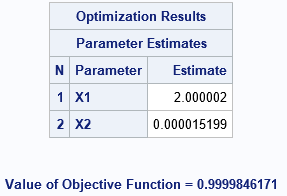The call to the NLPNMS subroutine generates a lot of output (because optn=4). The final parameter estimates are shown. The routine estimates the optimal parameters as (x,y) = (2.000002, 0.000015), which is very close to the exact optimal value (x,y)=(2,0). The objective function at the estimated parameters is within 1.5E-5 of the true maximum.

Visualizing the iteration path towards an optimum

I intentionally chose the initial guess for this problem to be (x0,y0)=(-2,0), which is diametrically opposite the true value in the annular region. I wanted to see how the optimization algorithm traverses the annular region. I knew that every partial solution would be within the feasible region, but I suspected that it might be possible for the iterates to "jump across" the hole in the middle of the annulus. The following SAS statements visualize the iteration history of the Nelder-Mead method as it iterates towards an optimal solution:

```data IterPath2; set IterPath; Iteration = ' '; if _N_ IN (1,5,6,7,9) then Iteration = put(_N_,2.); /* label certain step numbers */ format Iteration 2.; run;   data Path; set Viz IterPath2; run;   title "Objective Function and Solution Path"; title2 "Nelder-Mead Simplex Method"; proc sgplot data=Path noautolegend; xaxis grid; yaxis grid; scatter x=x y=y / markerattrs=(symbol=SquareFilled size=4) colorresponse=ObjFunc colormodel=ThreeColorRamp; polygon ID=R x=xx y=yy / lineattrs=(thickness=3); series x=x1 y=x2 / markers datalabel=Iteration datalabelattrs=(size=8) markerattrs=(size=5); gradlegend; run;```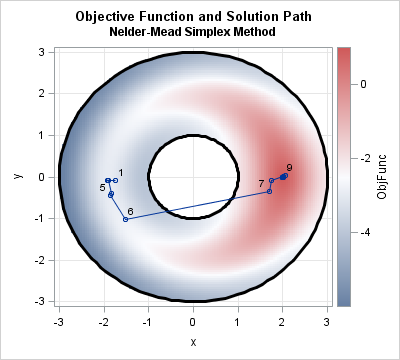For this initial guess and this optimization algorithm, the iteration path "jumps across" the hole in the annulus on the seventh iteration. For other initial guesses or methods, the iteration might follow a different path. For example, if you change the initial guess and use the quasi-Newton method, the optimization requires many more iterations and follows the "ridge" near the circle of radius 2, as shown below:
z0 = {-2 -0.1}; /* initial guess */
call NLPQN(rc,xOpt,"ObjFunc",z0,optn) nlc="NLConstr"; /* solve optimization */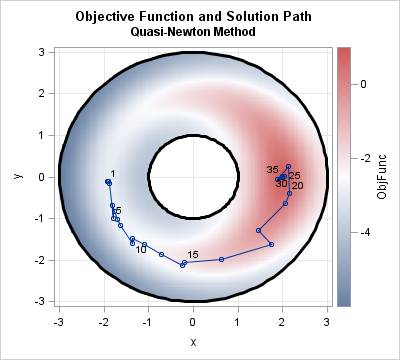Nonlinear constraints with PROC OPTMODEL

If you have access to SAS/OR software, PROC OPTMODEL provides a syntax that is compact and readable. For this problem, you can use a single CONSTRAINT statement to specify both the inner and outer bounds of the annular constraints. The following call to PROC OPTMODEL uses an interior-point method to compute a solution that numerically equivalent to (x,y) = (-2, 0):

```proc optmodel; var x, y; max f = cos(constant('pi')*sqrt(x^2+y^2)) - sqrt((x-2)^2+(y-0)^2); constraint 1 <= x^2 + y^2 <= 9; /* define constraint region */ x = -2; y = -0.1; /* initial guess */ solve with NLP / maxiter=25; print x y; quit;```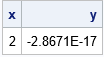Summary

In summary, you can use SAS software to solve nonlinear optimization problems that are unconstrained, bound-constrained, linearly constrained, or nonlinearly constrained. This article shows how to solve a nonlinearly constrained problem by using SAS/IML or SAS/OR software. In SAS/IML, you have to specify the number of nonlinear constraints and write a function that can evaluate a parameter value and return a vector of positive numbers if the parameter is in the constrained region.

The post Optimization with nonlinear constraints in SAS appeared first on The DO Loop.In the oil industry you can make or lose money based on how good your forecasts are, so I’ve pulled together six papers that discuss different ways in which you can leverage analytics to optimize your output and more accurately predict your production performance. Written by employees at oil and [...]

Data analysts often fit a probability distribution to data. When you have access to the data, a common technique is to use maximum likelihood estimation (MLE) to compute the parameters of a distribution that are "most likely" to have produced the observed data. However, how can you fit a distribution if you do not have access to the data?

This question was asked by a SAS programmer who wanted to fit a gamma distribution by using sample quantiles of the data. In particular, the p[rogrammer said, "we have the 50th and 90th percentile" of the data and "want to find the parameters for the gamma distribution [that fit] our data."

This is an interesting question. Recall that the method of moments uses sample moments (mean, variance, skewness,...) to estimate parameters in a distribution. When you use the method of moments, you express the moments of the distribution in terms of the parameters, set the distribution's moments equal to the sample moments, and solve for the parameter values for which the equation is true.

In a similar way, you can fit a distribution matching quantiles: Equate the sample and distributional quantiles and solve for the parameters of the distribution. This is sometimes called quantile-matching estimation (QME). Because the quantiles involve the cumulative distribution function (CDF), the equation does not usually have a closed-form solution and must be solved numerically.

Fit a two-parameter distribution from two quantiles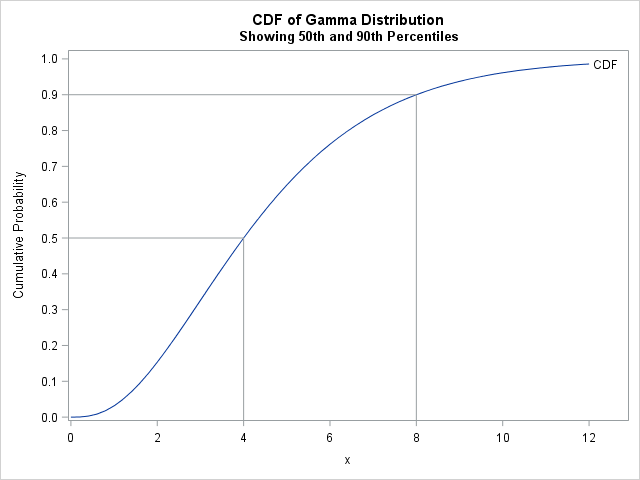To answer the programmer's question, suppose you do not have the original data, but you are told that the 50th percentile (median) of the data is x = 4 and the 90th percentile is x = 8. You suspect that the data are distributed according to a gamma distribution, which has a shape parameter (α) and a scale parameter (β). To use quantile-matching estimation, set F(4; α, β) = 0.5 and F(8; α, β) = 0.9, where F is the cumulative distribution of the Gamma(α, β) distribution. You can then solve for the values of (α, β) that satisfy the equations. You will get a CDF that matches the quantiles of the data, as shown to the right.

I have previously written about four ways to solve nonlinear equations in SAS. One way is to use PROC MODEL, as shown below:

```data initial; alpha=1; beta=1; /* initial guess for finding root */ p1=0.5; X1 = 4; /* eqn for 1st quantile: F(X1; alpha, beta) = p1 */ p2=0.9; X2 = 8; /* eqn for 2nd quantile: F(X2; alpha, beta) = p2 */ run;   proc model data=initial; eq.one = cdf("Gamma", X1, alpha, beta) - p1; /* find root of eq1 */ eq.two = cdf("Gamma", X2, alpha, beta) - p2; /* and eq2 */ solve alpha beta / solveprint out=solved outpredict; run;quit;   proc print data=solved noobs; var alpha beta; run;```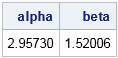The output indicates that the parameters (α, β) = (2.96, 1.52) are the values for which the Gamma(α, β) quantiles match the sample quantiles. You can see this by graphing the CDF function and adding reference lines at the 50th and 90th percentiles, as shown at the beginning of this section. The following SAS code creates the graph:

```/* Graph the CDF function to verify that the solution makes sense */ data Check; set solved; /* estimates of (alpha, beta) from solving eqns */ do x = 0 to 12 by 0.2; CDF = cdf("gamma", x, alpha, beta); output; end; run;   title "CDF of Gamma Distribution"; title2 "Showing 50th and 90th Percentiles"; proc sgplot data=Check; series x=x y=CDF / curvelabel; dropline y=0.5 X=4 / dropto=both; /* first percentile */ dropline y=0.9 X=8 / dropto=both; /* second percentile */ yaxis values=(0 to 1 by 0.1) label="Cumulative Probability"; xaxis values=(0 to 12 by 2); run;```

Least squares estimates for matching quantiles

The previous section is relevant when you have as many sample quantiles as parameters. If you have more sample quantiles than parameters, then the system is overconstrained and you probably want to compute a least squares solution. If there are m sample quantiles, the least squares solution is the set of parameters that minimizes the sum of squares Σim (piF(xi; α, β))2.

For example, the following DATA step contains five sample quantiles. The observation (p,q) = (0.1, 1.48) indicates that the 10th percentile is x=1.48. The second observation indicates that the 25th percentile is x=2.50. The last observation indicates that the 90th percentile is x=7.99. You can use PROC NLIN to find a least squares solution to the quantile-matching problem, as follows:

```data SampleQntls; input p q; /* p is cumul probability; q is p_th sample quantile */ datalines; 0.1 1.48 0.25 2.50 0.5 4.25 0.75 6.00 0.9 7.99 ;   /* least squares fit of parameters */ proc nlin data=SampleQntls /* sometimes the NOHALVE option is useful */ outest=PE(where=(_TYPE_="FINAL")); parms alpha 2 beta 2; bounds 0 < alpha beta; model p = cdf("Gamma", q, alpha, beta); run;   proc print data=PE noobs; var alpha beta; run;```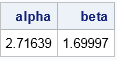The solution indicates the parameter values (α, β) = (2.72, 1.70) minimize the sum of squares between the observed and theoretical quantiles. The following graph shows the observed quantiles overlaid on the CDF of the fitted Gamma(α, β) distribution. Alternatively, you can graph the quantile-quantile plot of the observed and fitted quantiles.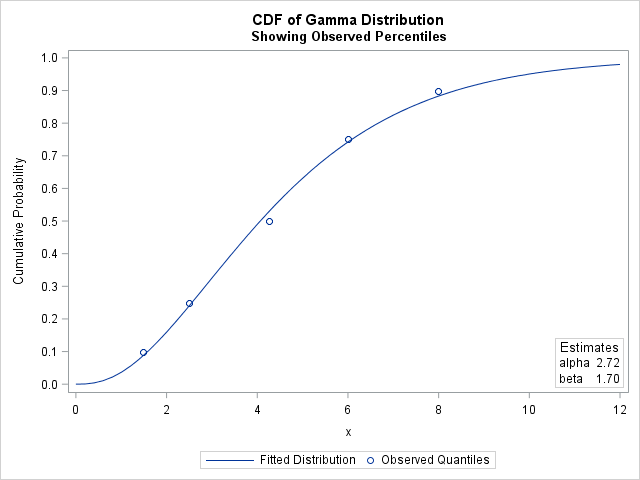Weighted least squares estimates for matching quantiles

For small samples, quantiles in the tail of a distribution have a large standard error, which means that the observed quantile might not be close to the theoretical quantile. One way to handle that uncertainty is to compute a weighted regression analysis where each sample quantile is weighted by the inverse of its variance. According to Stuart and Ord (Kendall’s Advanced Theory of Statistics, 1994, section 10.10), the standard error of the p_th sample quantile in a sample of size n is σ2 = p(1-p) / (n fp)2), where ξp is the p_th quantile of the distribution and f is the probability density function.

In PROC NLIN, you can perform weighted analysis by using the automatic variable _WEIGHT_. The following statements define the variance of the p_th sample quantile and define weights equal to the inverse variance. Notice the NOHALVE option, which can be useful for iteratively reweighted least squares problems. The option eliminates the requirement that the weighted sum of squares must decrease at every iteration.

```/* weighted least squares fit where w[i] = 1/variance[i] */ proc nlin data=SampleQntls NOHALVE outest=WPE(where=(_TYPE_="FINAL")); parms alpha 2 beta 2; bounds 0 < alpha beta; N = 80; /* sample size */ xi = quantile("gamma", p, alpha, beta); /* quantile of distrib */ f = pdf("Gamma", xi, alpha, beta); /* density at quantile */ variance = p*(1-p) / (N * f**2); /* variance of sample quantiles */ _weight_ = 1 / variance; /* weight for each observation */ model p = cdf("Gamma", q, alpha, beta); run;```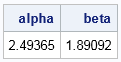The parameter estimates for the weighted analysis are slightly different than for the unweighted analysis. The following graph shows the CDF for the weighted estimates, which does not pass as close to the 75th and 90th percentiles as does the CDF for the unweighted estimates. This is because the PDF of the gamma distribution is relatively small for those quantiles, which causes the regression to underweight those sample quantiles.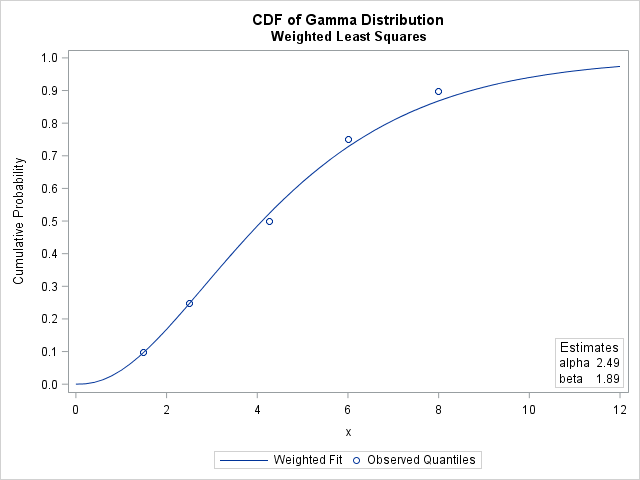In summary, this article shows how to use SAS to fit distribution parameters to observed quantiles by using quantile-matching estimation (QME). If the number of quantiles is the same as the number of parameters, you can numerically solve for the parameters for which the quantiles of the distribution equal the sample quantiles. If you have more quantiles than parameters, you can compute a least squares estimate of the parameters. Because quantile estimates in the tail of a distribution have larger uncertainty, you might want to underweight those quantiles. One way to do that is to run a weighted least squares regression where the weights are inversely proportional to the variance of the sample quantiles.

The post Fit a distribution from quantiles appeared first on The DO Loop.When you run an optimization, it is often not clear how to provide the optimization algorithm with an initial guess for the parameters. A good guess converges quickly to the optimal solution whereas a bad guess might diverge or require many iterations to converge. Many people use a default value (such as 1) for all initial guesses. I have previously written about other ways to obtain an initial guess for an optimization.

In the special case of maximum likelihood estimation, you use a trick to provide a good guess to the optimization algorithm. For maximum likelihood estimation, the objective function is the log-likelihood function of a distribution. Consequently, you can use the method of moments to provide the initial guess for the parameters, which often results in fast convergence to the maximum likelihood estimates. The faster convergence can save you considerable time when you are analyzing thousands of data sets, such as during a simulation study.

The method of moments

Suppose you believe that some data can be modeled by a certain distribution such as a lognormal, Weibull, or beta distribution. A common way to estimate the distribution parameters is to compute the maximum likelihood estimates (MLEs). These are the values of the parameters that are "most likely" to have generated the observed data. To compute the MLEs you first construct the log-likelihood function and then use numerical optimization to find the parameter values that maximize the log-likelihood function.

The method of moments is an alternative way to fit a model to data. For a k-parameter distribution, you write the equations that give the first k central moments (mean, variance, skewness, ...) of the distribution in terms of the parameters. You then replace the distribution's moments with the sample mean, variance, and so forth. You invert the equations to solve for the parameters in terms of the observed moments.

For example, suppose you believe that some data are distributed according to a two-parameter beta distribution. If you look up the beta distribution on Wikipedia, you will see that the mean and variance of a Beta(a, b) distribution can be expressed in terms of the two parameters as follows: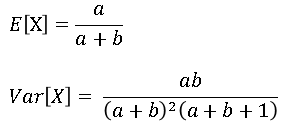The first equation represents the expected (mean) value of a beta-distributed random variable X. The second equation represents the variance. If the sample mean of the data is m and the sample variance is v, you can "plug in" these values for the left-hand side of the equations and solve for the values of a and b that satisfy the equations. Solving the first equation for a yields a = b m / (1 - m). If you substitute that expression into the second equation and solve for b, you get b = m - 1 + (m/v)(1 - m)2. Those equations give the parameter estimates from the method of moments.

Maximum likelihood estimation: Using an arbitrary guess

The method of moments can lead to improved convergence when fitting a distribution. For comparison, first consider what happens if you use an arbitrary initial guess for the optimization, such as a = b = 1. The following statements use PROC NLMIXED to compute maximum likelihood estimates for the parameters of the beta distribution. (You can read about how to use PROC NLMIXED to construct MLEs. You can also compute MLEs by using SAS/IML.)

```data Beta; input x @@; datalines; 0.58 0.23 0.41 0.69 0.64 0.12 0.07 0.19 0.47 0.05 0.76 0.18 0.28 0.06 0.34 0.52 0.42 0.48 0.11 0.39 0.24 0.25 0.22 0.05 0.23 0.49 0.45 0.29 0.18 0.33 0.09 0.16 0.45 0.27 0.31 0.41 0.30 0.19 0.27 0.57 ;   /* Compute parameter estimates for the beta distribution */ proc nlmixed data=Beta; parms a=1 b=1; /* use arbitrary initial guesses */ bounds a b > 0; LL = logpdf("beta", x, a, b); /* log-likelihood function */ model x ~ general(LL); run;```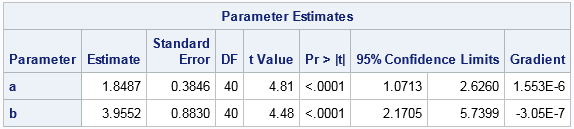The MLEs are a = 1.8487 and b = 3.9552. The "Iteration History" table (not shown) shows that 8 iterations of a quasi-Newton algorithm were required to converge to the MLEs, and the objective function (the LOGPDF function) was called 27 times during the optimization.

The following graph shows a histogram of the data overlaid with a Beta(a=1.85, b=3.96) density curve. The curve fits the data well.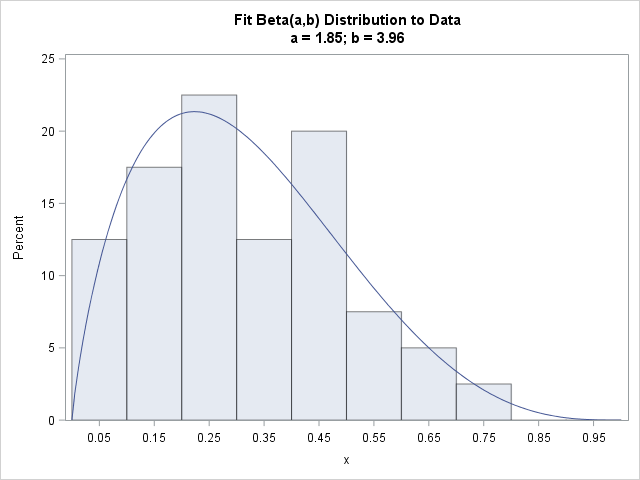Maximum likelihood estimation: Using method-of-moments for guesses

If you use the method-of-moments to provide an initial guess on the PARMS statement, the quasi-Newton algorithm might converge in fewer iterations. The following statements use PROC MEANS to compute the sample mean and variance, the use the DATA step to compute the method-of-moments estimates, as follows:

```/* output mean and variance */ proc means data=Beta noprint; var x; output out=Moments mean=m var=v; /* save sample moments */ run;   /* use method of moments to estimate parameters for the beta distribution */ data ParamEst; retain a b; /* set order of output variables */ set Moments; b = m*(1-m)**2/v + m - 1; /* estimate parameters by using sample moments */ a = b*m / (1-m); keep a b; run;```

The method of moments estimates are a = 1.75 and b = 3.75, which are close to the MLE values. The DATA= option in the PARMS statement in PROC NLMIXED enables you to specify a data set that contains starting values for the parameters. The following call to PROC NLMIXED is exactly the same as the previous call except that the procedure uses the initial parameter values from the ParamEst data set:

```proc nlmixed data=Beta; parms / data=ParamEst; /* use initial guesses from method of moments */ bounds a b > 0; LL = logpdf("beta", x, a, b); /* log-likelihood function */ model x ~ general(LL); run;```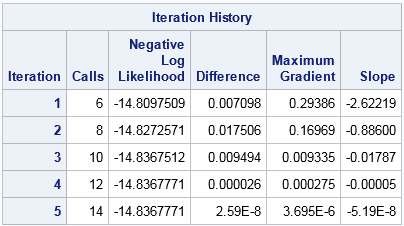The MLEs from this call (not shown) are exactly the same as before. However, the "Iteration History" table indicates that quasi-Newton algorithm converged in 5 iterations and required only 14 calls the objective function. This compares with 8 iterations and 27 calls for the previous computation. Thus the method of moments resulted in about half as many functional evaluations. In my experience this example is typical: Choosing initial parameters by using the method of moments often reduces the computational time that is required to obtain the MLEs.

It is worth mentioning that the PARMS option in PROC NLMIXED supports two nice features:

• The DATA= option accepts both wide and long data sets. The example here is wide: there are k variables that supply the values of the k parameters. You can also specify a two-variable data set with the names "Parameter" and "Estimate."
• The BYDATA option enables you to specify initial guesses for each BY group. In simulation studies, you might use BY-group processing to compute the MLEs for thousands of samples. The BYDATA option is essential to improving the efficiency of a large simulation study. It can also help ensure that the optimization algorithm converges when the parameters for the samples range over a large set of values.

Summary and cautions

In summary, this article shows how to use the method of moments to provide a guess for optimizing a log-likelihood function, which often speeds up the time required to obtain the MLEs. The method requires that you can write the equations that relate the central moments of a distribution (mean, variance, ...) to the parameters for the distribution. (For a distribution that has k parameters, use the equations for the first k moments.) You then "plug in" the sample moments and algebraically invert the equations to obtain the parameter values in terms of the sample moments. This is not always possible analytically; you might need to use numerical methods for part of the computation.

A word of caution. For small samples, the method of moments might produce estimates that do not make sense. For example, the method might produce a negative estimate for a parameter that must be positive. In these cases, the method might be useless, although you could attempt to project the invalid parameters into the feasible region.

The post The method of moments: A smart way to choose initial parameters for MLE appeared first on The DO Loop.Every fall, highways, backroads and neighborhood streets nationwide take on a noticeable yellow hue, as school buses carefully and methodically transport students back to school. In some areas, including Boston, this massive transportation exercise can present a number of challenges. Boston Public Schools (BPS) provided transportation for 25,000 students via [...]

Did you know that you can get SAS to compute symbolic (analytical) derivatives of simple functions, including applying the product rule, quotient rule, and chain rule? SAS can form the symbolic derivatives of single-variable functions and partial derivatives of multivariable functions. Furthermore, the derivatives are output in a form that can be pasted into a SAS program. The trick is to use PROC NLIN with the LIST option.

In SAS/IML, the nonlinear optimization routines will use analytical derivatives if they are provided; otherwise, they will automatically generate numerical finite-difference approximations to the derivatives. I rarely specify derivatives because it can be time-consuming and prone to error. But recently I realized that PROC NLIN and other SAS procedures automatically generate symbolic derivatives, and you can "trick" the procedure into displaying the derivatives so that they can be used in other applications. To get PROC NLIN to output symbolic derivatives, do the following:

• Create a data set to use for the DATA= option of PROC NLIN.
• List the variables that you want to take derivatives of on the PARMS statement. In open code, assign a value to constants that appear in the function.
• Specify the function to differentiate on the MODEL statement.

Symbolic derivatives of functions of one variable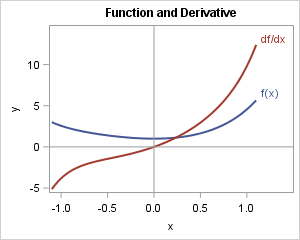Here is a one-variable example. Suppose you want to take the derivative of
f(x) = x3 + x sin(x) + exp( x2 ).
The function and its derivative is shown to the right.

The following SAS statements create a "fake" data set that defines a variable named F. The call to PROC NLIN sets up the problem. The LIST option on the PROC NLIN statement generates the 'ProgList' table, which contains the derivative function:

```data _dummy; f = 1; /* name of function */ run;   proc nlin data=_dummy list; parms x=0; /* list variables */ model f = x**3 + x*sin(x) + exp(x**2); /* define function */ ods select ProgList; /* output symbolic derivatives */ run;```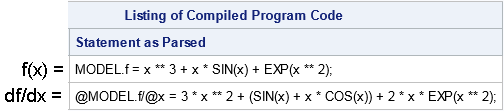The output shows the expressions for the function ("MODEL.f") and the derivative with respect to x. The derivative df/dx is written as "@MODEL.f/@x", where the symbol '@' is used for the 'd'. You can see that SAS took the simple derivative of the x3 term, applied the product rule to the x*sin(x) term, and applied the chain rule to the exp( x2 ) term. You can also see that the expressions are written in SAS notation, with "**" indicating the power operator. SAS functions are written in upper case (SIN, COS, and EXP).

Symbolic partial derivatives of multivariate functions

In exactly the same way, you can obtain partial derivatives. In a partial derivative, all variables are held constant except one. Suppose you have the function for the normal density,
f(x) = 1/(sqrt(2 π) σ) exp( -(x - μ)**2 / (2 σ**2) )
Suppose that you want to compute the partial derivatives ∂f/∂μ and ∂f/∂σ. To compute these derivatives, list μ and σ on the PARMS statement and assign values to the other symbols (x and π) that appear in the function. It does not matter what value you assign to the x and π, you are just preventing PROC NLIN from complaining that there are unassigned variables. You could assign the value 1 to both variables, but I prefer to tell the procedure that π equals 3.14. In the following program, note that PROC NLIN reuses the fake data set that defines the F variable:

```proc nlin data=_dummy list; parms mu=0 sigma=1; /* list variables */ x=1; pi = constant('pi'); /* assign arbitrary values to constants */ model f = exp( -(x-mu)**2 / (2*sigma**2) ) / (sqrt(2*pi)*sigma); /* define function */ ods select ProgList; /* output symbolic derivatives */ run;```The derivative with respect to σ requires using the chain rule and the quotient rule. Notice that the derivative includes a call to the original function ("MODEL.f"). Notice also that there is repetition of various expressions such as -(x - μ)**2 / (2 σ**2). If you copy and paste these expressions into a SAS program that computes the derivative, the computation will be slightly inefficient because the program will evaluate the same expression multiple times. One way to improve the efficiency is to collect sub-expressions into a temporary variable, as shown in the next section.

Sub-expressions and the chain rule

An experienced statistician will recognize that the expression z = (x - μ) / σ arises naturally as the standardized variable. Using such an expression might simplify the derivatives.

The following program computes the same derivatives as before, except this time the programmer defines the expression z = (x - μ) / σ and uses z in the function. When SAS takes the derivative, it computes dz/dμ and dz/dσ as part of the computation, as shown below:

```proc nlin data=_dummy list; parms mu=0 sigma=1; /* list variables */ x=1; pi = constant('pi'); /* assign arbitrary values to constants */ const = sqrt(2*pi); z = (x-mu) / sigma; /* intermediate expression */ model f = exp(-z**2 / 2) / (const*sigma); /* define function */ ods select ProgList; /* output symbolic derivatives */ run;```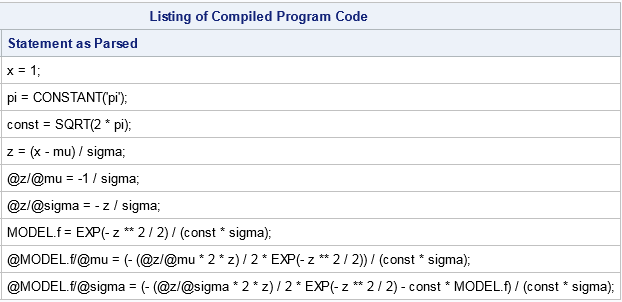Notice that the derivatives use several sub-expressions such as dz/dμ and dz/dσ. This computation is more efficient than the previous because it reuses previously computed quantities. For another example, define f1 = 1 / sqrt(2*pi*sigma) and f2 = exp(-z**2 / 2) and define the MODEL as f = f1*f2. You will see that each term in the derivative is efficiently evaluated in terms of previously expressions.

Discussion of symbolic derivatives

As I said previously, I do not usually specify analytical derivatives for my optimizations. I find that numerical derivatives (which are automatically computed) are fast and accurate for 99% of the optimizations that I perform.

Furthermore, some of the objective functions that I optimize do not have analytical derivatives. Others are matrix computations for which it would be difficult and time-consuming to write down the analytical derivative. (For an example, see the article on the derivative of the bivariate normal cumulative distribution.)

If you decide to specify an analytical derivative in SAS, make sure that the derivative is correct and efficient. The technique in this article will help to ensure that the derivative is correct. By strategically defining sub-expressions as in the previous section, you can help SAS generate derivatives that are computationally efficient.

Be aware that the derivatives are generated by applying the chain rule, product rule, and quotient rule without any algebraic simplifications of the result. Thus the result can be unnecessarily complicated. For an example, ask SAS to display the derivative of log( (1+x)/(1-x) ) / 2. The naive result is complicated. If you rewrite the function as log(1+x)/2 - log(1-x)/2, then the derivative is much simpler. However, in neither case will SAS simplify the derivative to obtain 1 / (1-x**2).

For more of my thoughts on using derivatives in SAS, see "Two hints for specifying derivatives." What about your thoughts? What do you think about using PROC NLIN to generate automatic derivatives of simple functions? Do you think this technique will be useful to you?

The post Symbolic derivatives in SAS appeared first on The DO Loop.